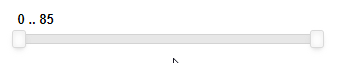# How to create a RangeSlider with non-uniform step distance?

Background: I have a group of datasets showing population data for various age groups: [0, 1, 5, 10, 15, 20… 75, 80] where the number refers to the lower bound of the age group and the next number the upper bound, so 0 means 0<=x<1, 1 means 1<=x<5 and from there on every number means the next 5 years, except for the 80 which means 80<=x.

Task: I need to have a slider where the user can select between these numbers. If all the numbers would be multiples of 5, I could use `step`, but 0 and 1 are different. If the users could choose anything (like 7), I could just use `step=1`, and then show them the closest, but I have to force them to pick a valid value.

My best idea so far is to index the groups from 0, and then use PrintfTickFormatter to display the real value (so if 3 is selected, 10 should be displayed) AND to do that at the same time when dealing with the picked value.

But this feels like a suboptimal hack where the saved and displayed values are and should be the same, I’m just doing a two-way conversion every time between them. Also, this will force a uniform distance between the ticks which may or may not be desired. (For me it will depend on the available space in the end: if it would be a wide slider, a non-uniform distribution would be better. If it would be a short one then uniform distribution actually would be more useful.)

DiscreteSlider does not fit your need?

``````import panel as pn
pn.extension()

s = pn.widgets.DiscreteSlider(options=[0,1,5,10,15,20,75,80])
s
``````

Thanks, I did not find that as I was only checking the numeric types, my bad.
Another error on my part that I did not specify that I need a range slider. The users should be able to select the limits of a composite age group, like [1, 15], I just thought this would be an irrelevant detail as both numeric slider has a RangeSlider version. But it seems I would need a DiscreteRangeSlider that does not exists, so I should either use my workaround or use two DiscreteSliders, is that correct?

Indeed there is no discreteRangeSlider
A base for the implementation could be:

``````import panel as pn
pn.extension()
import param
from bokeh.models import FuncTickFormatter
class DiscreteRangeSlider(pn.widgets.RangeSlider):
options = param.List()
_rename = {"options":None}
def __init__(self, options, **params):

params.update({
"start": 0,
"end": len(options)-1,
"options": options
})
if "value" not in params:
params.update({"value": (options, options[-1])})
formatter = FuncTickFormatter(args={"options":options}, code="""
return options[tick]
""")
params.update({"format": formatter})
super().__init__(**params)

def _process_property_change(self, msg):
if "value" in msg:
value = msg["value"]
msg["value"] = (self.options[value], self.options[value])
if "value_throttled" in msg:
value_throttled = msg["value_throttled"]
msg["value_throttled"] = (self.options[value_throttled], self.options[value_throttled])
return msg

def _process_param_change(self, msg):
msg = super()._process_param_change(msg)
if "value" in msg:
value = msg["value"]
msg["value"] = (self.options.index(value),self.options.index(value))
return msg

DiscreteRangeSlider(value=(0,1), options=[0,1,5,25,85])
``````

2 Likes

Jesus, did you just develop a custom widget for me on the fly???That looks exactly what I need, but I did not expect this. Thank you very much, this is amazing!

1 Like

It’s a rough implementation but I think a DiscreteRangeSlider is a legitim demand to open on the github tracker Issues · holoviz/panel · GitHub

1 Like

I’ve managed to try it out. It worked almost perfectly with one issue (which also caused the managed function to freeze):When I displayed the tick value, it was like 2.9999999999999996 and 4.000000000001, so some float point problem is going on in the background. I resolved it by casting both the tick value in javascript, and the returned value in python to int, but most probably it would be better in the final version if the options would stay int. I will submit a feature request and mention this there too, for now this is perfectly fine.

1 Like